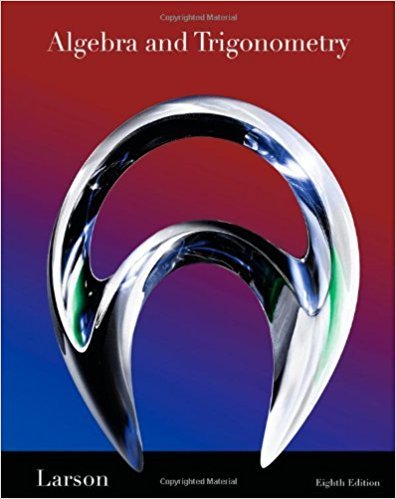×
×

# Solutions for Chapter 3.3: Polynomial and Synthetic Division## Full solutions for Algebra and Trigonometry | 8th Edition

ISBN: 9781439048474Solutions for Chapter 3.3: Polynomial and Synthetic Division

Solutions for Chapter 3.3
4 5 0 404 Reviews
17
3
##### ISBN: 9781439048474

Chapter 3.3: Polynomial and Synthetic Division includes 100 full step-by-step solutions. Algebra and Trigonometry was written by and is associated to the ISBN: 9781439048474. Since 100 problems in chapter 3.3: Polynomial and Synthetic Division have been answered, more than 47336 students have viewed full step-by-step solutions from this chapter. This textbook survival guide was created for the textbook: Algebra and Trigonometry, edition: 8. This expansive textbook survival guide covers the following chapters and their solutions.

Key Math Terms and definitions covered in this textbook
• Affine transformation

Tv = Av + Vo = linear transformation plus shift.

• Column space C (A) =

space of all combinations of the columns of A.

• Condition number

cond(A) = c(A) = IIAIlIIA-III = amaxlamin. In Ax = b, the relative change Ilox III Ilx II is less than cond(A) times the relative change Ilob III lib II· Condition numbers measure the sensitivity of the output to change in the input.

• Cramer's Rule for Ax = b.

B j has b replacing column j of A; x j = det B j I det A

• Free columns of A.

Columns without pivots; these are combinations of earlier columns.

• Hermitian matrix A H = AT = A.

Complex analog a j i = aU of a symmetric matrix.

• Hessenberg matrix H.

Triangular matrix with one extra nonzero adjacent diagonal.

• Incidence matrix of a directed graph.

The m by n edge-node incidence matrix has a row for each edge (node i to node j), with entries -1 and 1 in columns i and j .

• Kronecker product (tensor product) A ® B.

Blocks aij B, eigenvalues Ap(A)Aq(B).

• Krylov subspace Kj(A, b).

The subspace spanned by b, Ab, ... , Aj-Ib. Numerical methods approximate A -I b by x j with residual b - Ax j in this subspace. A good basis for K j requires only multiplication by A at each step.

• Markov matrix M.

All mij > 0 and each column sum is 1. Largest eigenvalue A = 1. If mij > 0, the columns of Mk approach the steady state eigenvector M s = s > O.

• Matrix multiplication AB.

The i, j entry of AB is (row i of A)·(column j of B) = L aikbkj. By columns: Column j of AB = A times column j of B. By rows: row i of A multiplies B. Columns times rows: AB = sum of (column k)(row k). All these equivalent definitions come from the rule that A B times x equals A times B x .

• Minimal polynomial of A.

The lowest degree polynomial with meA) = zero matrix. This is peA) = det(A - AI) if no eigenvalues are repeated; always meA) divides peA).

• Normal matrix.

If N NT = NT N, then N has orthonormal (complex) eigenvectors.

• Nullspace N (A)

= All solutions to Ax = O. Dimension n - r = (# columns) - rank.

• Pivot columns of A.

Columns that contain pivots after row reduction. These are not combinations of earlier columns. The pivot columns are a basis for the column space.

• Pseudoinverse A+ (Moore-Penrose inverse).

The n by m matrix that "inverts" A from column space back to row space, with N(A+) = N(AT). A+ A and AA+ are the projection matrices onto the row space and column space. Rank(A +) = rank(A).

• Rayleigh quotient q (x) = X T Ax I x T x for symmetric A: Amin < q (x) < Amax.

Those extremes are reached at the eigenvectors x for Amin(A) and Amax(A).

• Schwarz inequality

Iv·wl < IIvll IIwll.Then IvTAwl2 < (vT Av)(wT Aw) for pos def A.

• Special solutions to As = O.

One free variable is Si = 1, other free variables = o.

×